# KPI Partners Blog

### by Kurt Wolff

An OBIEE dashboard designer recently asked how to turn a prompt for a single month into a filter for a range of months. For example, if the user selects “Aug” in the prompt, the results should include not just August but also the three months preceding and following August (i.e. May through Nov).

One way to do this is to take advantage of sequence numbers for months (giving an easy way to calculate a range of months) and presentation variables.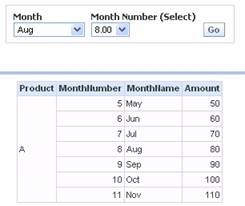Here’s the prompt setup.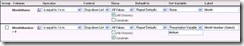Notice three things. The user’s selection of MonthNumber sets the value of a presentation variable called MoNum. Notice also that the formula for MonthNumber is not simply the column name itself but an expression: MonthNumber + 0. Finally, note that MonthNumber is constrained so that it is effectively set by the user’s choice of MonthName.

For the query itself, the tricky part is setting the filter. There are two things to note here. The first is the use of “SQL Expressions” in the filter. The second is the syntax for the presentation variable in these SQL expressions.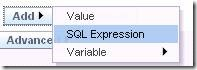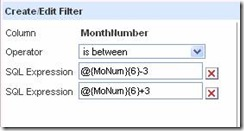Of course, instead of a hardcoded value of 3 in these expressions, you could also use another presentation variable. To illustrate, I changed the prompt to have an edit box that enables the user to set a presentation variable called “Range”.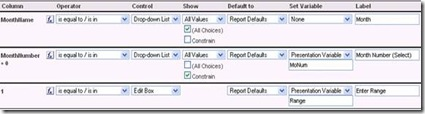Notice here that the “Column” is not a column at all but simply the number 1. To generate this, click any numeric column in the left panel, then change the formula to 1.

On the dashboard, the prompt and query work together as shown here.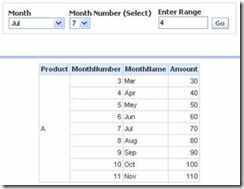The set up for the filter on the query includes this Range variable with a default value of 1. (This 1 is not connected at all to the fact that 1 was used in the formula in the prompt).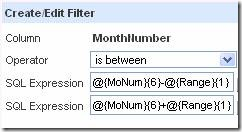Finally, you may be wondering why the formula for MonthNumber was set to MonthNumber+0 in the prompt. The reason is that if the formula in the prompt is the same as the formula for the column in the query, then the MonthNumber itself gets set as the filter. Here’s an example. The user selects May (month number 5) with a range of 2. The query shows the results for May only.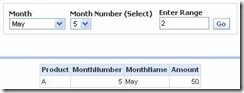Looking at how the query was modified by the prompt, you can see that the filter used just the value of MonthNumber in the prompt. The presentation variables were ignored.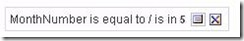Tags: Kurt Wolff, Oracle BI, Blog